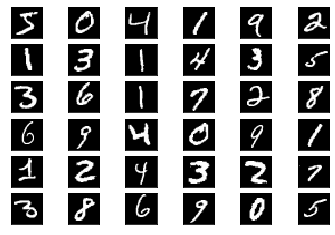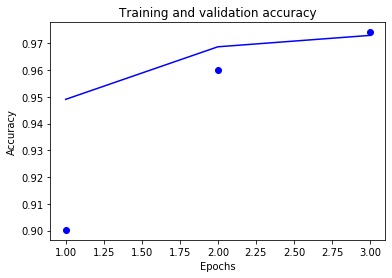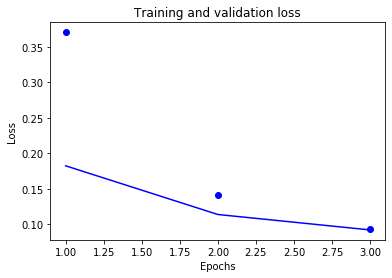Learn data science and machine learning by building real-world projects on Jovian

## Handwritten Digit Recognition

Objective: Classify handwritten digits from the MNIST dataset by training a convolutional neural network (CNN) using the Keras deep learning library.

### Data Preparation

We begin by downloading the data and creating training & validation sets. Keras has inbuilt helper functions to do this.

In :
``````from keras.datasets import mnist
(train_images, train_labels), (test_images, test_labels) = mnist.load_data()
``````
```Using TensorFlow backend. ```

Each sample is a 28px x 28 px image, flattened out a vector of length 784 i.e. 28x28.

In :
``train_images.shape, train_labels``
Out:
``((28, 28), 5)``
In :
``````import jovian

jovian.log_dataset({
'from': '2019-01-01',
'to': '2019-02-01',
'features': ['created_at']
})
``````
```[jovian] Dataset logged. ```

Let's take a look at some sample images from the training set, by plotting them in a grid.

In :
``````%matplotlib inline
import matplotlib.pyplot as plt

grid_size = 6
f, axarr = plt.subplots(grid_size, grid_size)
for i in range(grid_size):
for j in range(grid_size):
ax = axarr[i, j]
ax.get_xaxis().set_visible(False)
ax.get_yaxis().set_visible(False)
ax.imshow(train_images[i * grid_size + j], cmap='gray')``````We're going to apply the following preprocessing steps:

1. Reshape the image vectors into 28x28 matrices
2. Separate 20% of the training set to create a vaidation set
3. Conver the numeric labels into one-hot vectors
In :
``````train_images = train_images.reshape((60000, 28, 28, 1))
train_images = train_images.astype('float32') / 255
test_images = test_images.reshape((10000, 28, 28, 1))
test_images = test_images.astype('float32') / 255

from keras.utils import to_categorical

partial_train_images = train_images[:45000]
partial_train_labels = train_labels[:45000]

validation_images = train_images[45000:]
validation_labels = train_labels[45000:]

partial_train_labels = to_categorical(partial_train_labels)
validation_labels = to_categorical(validation_labels)
test_labels = to_categorical(test_labels)
``````

### Model & Training

Now we're ready to define a simple CNN model.

In :
``````input_shape = (28,28,1)
num_classes = 10``````
In :
``````from keras.models import Sequential
from keras.layers import Conv2D, MaxPooling2D, Flatten, Dense

model = Sequential()

model.summary()
``````
```WARNING:tensorflow:From /usr/local/anaconda3/envs/keras-mnist-jovian/lib/python3.7/site-packages/tensorflow/python/framework/op_def_library.py:263: colocate_with (from tensorflow.python.framework.ops) is deprecated and will be removed in a future version. Instructions for updating: Colocations handled automatically by placer. _________________________________________________________________ Layer (type) Output Shape Param # ================================================================= conv2d_1 (Conv2D) (None, 26, 26, 32) 320 _________________________________________________________________ max_pooling2d_1 (MaxPooling2 (None, 13, 13, 32) 0 _________________________________________________________________ flatten_1 (Flatten) (None, 5408) 0 _________________________________________________________________ dense_1 (Dense) (None, 10) 54090 ================================================================= Total params: 54,410 Trainable params: 54,410 Non-trainable params: 0 _________________________________________________________________ ```
In :
``````from jovian.callbacks.keras import JovianKerasCallback

model.compile(
optimizer='rmsprop', loss='categorical_crossentropy', metrics=['accuracy'],
)
``````
In [ ]:
``log_hyperparams``
In :
``````history = model.fit(
partial_train_images,

partial_train_labels,
epochs=1,
batch_size=128,
callbacks=[JovianKerasCallback()],
validation_data=(validation_images, validation_labels))
``````
```Train on 45000 samples, validate on 15000 samples Epoch 1/1 45000/45000 [==============================] - 26s 569us/step - loss: 0.0748 - acc: 0.9783 - val_loss: 0.0814 - val_acc: 0.9759 ```
In :
``````import matplotlib.pyplot as plt

acc = history.history['acc']
loss = history.history['loss']
val_acc = history.history['val_acc']
val_loss = history.history['val_loss']

epochs = range(1, len(acc) + 1)

plt.plot(epochs, acc, 'bo', label='Training acc')
plt.plot(epochs, val_acc, 'b', label='Validation acc')
plt.title('Training and validation accuracy')
plt.xlabel('Epochs')
plt.ylabel('Accuracy')
plt.margins(0.05)

plt.figure()

plt.plot(epochs, loss, 'bo', label='Training loss')
plt.plot(epochs, val_loss, 'b', label='Validation loss')
plt.title('Training and validation loss')
plt.xlabel('Epochs')
plt.ylabel('Loss')
plt.margins(0.05)

plt.show()
``````### Model Evaluation

In :
``test_loss, test_acc = model.evaluate(test_images, test_labels)``
```10000/10000 [==============================] - 1s 93us/step ```
In :
``````print('Test loss:', test_loss)
print('Test acc:', test_acc)``````
```Test loss: 0.07964395569600165 Test acc: 0.9751 ```

We can also save the trained model's weights to disk, so we won't need to train it again.

In :
``model.save('mnist-cnn.h5')``

### Save & Commit

In :
``!pip install jovian --upgrade``
```Requirement already up-to-date: jovian in /usr/local/anaconda3/envs/keras-mnist-jovian/lib/python3.7/site-packages (0.1.89) Requirement already satisfied, skipping upgrade: requests in /usr/local/anaconda3/envs/keras-mnist-jovian/lib/python3.7/site-packages (from jovian) (2.22.0) Requirement already satisfied, skipping upgrade: uuid in /usr/local/anaconda3/envs/keras-mnist-jovian/lib/python3.7/site-packages (from jovian) (1.30) Requirement already satisfied, skipping upgrade: pyyaml in /usr/local/anaconda3/envs/keras-mnist-jovian/lib/python3.7/site-packages (from jovian) (5.1) Requirement already satisfied, skipping upgrade: idna<2.9,>=2.5 in /usr/local/anaconda3/envs/keras-mnist-jovian/lib/python3.7/site-packages (from requests->jovian) (2.8) Requirement already satisfied, skipping upgrade: chardet<3.1.0,>=3.0.2 in /usr/local/anaconda3/envs/keras-mnist-jovian/lib/python3.7/site-packages (from requests->jovian) (3.0.4) Requirement already satisfied, skipping upgrade: certifi>=2017.4.17 in /usr/local/anaconda3/envs/keras-mnist-jovian/lib/python3.7/site-packages (from requests->jovian) (2019.3.9) Requirement already satisfied, skipping upgrade: urllib3!=1.25.0,!=1.25.1,<1.26,>=1.21.1 in /usr/local/anaconda3/envs/keras-mnist-jovian/lib/python3.7/site-packages (from requests->jovian) (1.25.3) ```
In :
``import jovian``
In [ ]:
``jovian.commit()``
```[jovian] Saving notebook.. ```
In [ ]:
`` ``